# Operator Theory

Also found in: Wikipedia.

## operator theory

[′äp·ə‚rād·ər ‚thē·ə·rē]
(mathematics)
The general qualitative study of operators in terms of such concepts as eigenvalues, range, domain, and continuity.

## Operator Theory

the branch of functional analysis that studies the properties of operators and the application of operators to the solution of various problems. The operator concept is one of the most general in mathematics.

Some examples of operators follow. (1) By assigning to each vector (ξ1, ξ23) a vector (ξʹ1, ξʹ2, ξʹ3) such that ξʹi = ai1ξ1 + ai2ξ;2+ ai3ξ;3 (i = 1, 2, 3; ai1, ai2, and ai3 are fixed numbers), we obtain an operator.

(2) The differentiation operation, or operator, D[f(t)] = fʹ (t) takes every differentiable function f(t) into its derivative fʹ t).

(3) The operation, or operator, of definite integration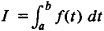takes every integrable function into a real number.

(4) By assigning to every function f(t) its product Φ (t) f(t) with a fixed function Φ(t), we obtain an operator.

General operator theory arose as a result of the development of such branches of classical analysis as the theory of integral equations and the solution of eigenfunction and eigenvalue problems for differential operators, for example, the Sturm-Liouville problem. Operator theory established close ties between these branches of mathematics and played an important role in their further development. Even before the general concept of an operator arose, operator methods were widely applied in the solution of various types of ordinary and partial differential equations. Operator theory provides the basic mathematical framework of quantum mechanics.

Operators in linear spaces. The most frequently encountered operators are those that act in normed linear spaces, particularly in function spaces. Such an operator is a mapping y = A(x) of a linear space R or part of it into a linear space R’, which may coincide with R. This class of operators encompasses such very important concepts as functions numerical-valued, linear transformations of a Euclidean space, and differential and integral operators (see below). Linear operators are the most widely studied class of operators and the most important from the standpoint of applications. An operator is called linear if Ax + β y) = α A(x) + β A(y) for any elements ξ and y of the space R and any numbers o and β. If the spaces R and R’ are normed and if the ratio ǀǀA(X) ǀǀ/ǀǀX ǀǀ of the norm of A(x) and the norm of x is bounded, then the linear operator A is said to be bounded and the least upper bound of the ratio ǀǀA(x)ǀǀ/ǀǀ(x)ǀǀ is called its norm. The boundedness of a linear operator is equivalent to its continuity, that is, A(xnA(x) as xnx. The differentiation operator defined in (2) is one of the most important examples of an unbounded, and consequently discontinuous, linear operator.

The four examples given above are linear operators. The following are also examples of linear operators:

(5) Let k(s, t) be a continuous function of two variables defined on the square αsb, αtb. The equation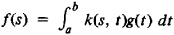defines a linear integral operator called the Fredholm operator.

(6) Let f(t) be a function that is absolutely integrable on the real line. The correspondence that associates to f(t) its Fourier transform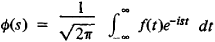is a linear operator. (7) The left-hand side of the linear differential equation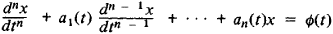may be regarded as the result of the application of an operator that associates to the function x(t) the function x (t). Such an operator is termed a linear differential operator. The simplest case of a linear differential operator is the differentiation operator.

The following are examples of nonlinear operators:

(8) Let A [f(t)] =f2(t). The operator thus defined is nonlinear.

(9) Let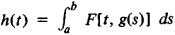where F is a bounded continuous function. The correspondence gh defined by this expression is a nonlinear integral operator.

Operations on operators. Let the operator

y = A(X)

be one-to-one. Then to each images there corresponds a unique preimage x. This correspondence is called the inverse operator and is denoted by

x = A-1(y)

Obtaining the inverse operator is equivalent to solving the equation y= A(x) for x, that is, the finding of an unknown preimage for a given image.

If A1 and A2 are two operators that map R into , then their sum A = A1 + A1 is (x). If the operator A1 maps R into R’ and A2 maps R’ into R”, then the result of the successive application of these operators is an operator that maps R into R” and is called the product A2A1 of the operators A1 and A2. If, in particular, we consider operators that map a linear space into itself, then the sum and product of two such operators are always defined. The result of η successive applications of the operator A is the nth power An of that operator. For example, the nth power of the differentiation operator is the n-fold differentiation operator Dn [f(t)] = fin)(t). The product λ A of an operator A and a number λ is defined by the formula

A)(x) = λA (x)

The operator E that maps every element ξ into itself is called the identity operator. The operator O that maps every element into zero is called the zero operator. The following equalities obviously hold for any A: AE = EA = A, and A + O = O + A = A. Furthermore, if A-1 exists, then A-1A = AA-1 = E. It should be noted, however, that in general for two arbitrary operators A and E the products AB and BA are not equal.

By means of the operations of operator addition, operator multiplication, and multiplication of an operator by a number, one may define polynomials of a linear operator and more complicated functions by using a suitably defined limit process. For example, if D is the differentiation operator, then eD denotes the operator defined by the formulawhich is valid for those f(t) for which the series on the right converges. For analytic functions the sum of this series equals f(t + 1); that is, eD is a shift operator mapping f(t) into f(t + 1).

Linear operators in Hilbert space. Operator theory has most fully been worked out for the case of linear operators in a Hilbert space. Let A be a bounded linear operator in a Hilbert space H. The complex number λ is called an eigenvalue of A if there exists a nonzero element x in H such that A(x) = λx; x is called an eigenvector of A belonging to the eigenvalue λ. A number λ is called a regular point of A if the operator (A —λE)-1 exists, is defined on all of H, and is bounded; the remaining values of λ are called the points of the spectrum of A. Every eigenvalue of A belongs to the spectrum; the set of eigenvalues of A is the point spectrum, and the rest of the spectrum is called the continuous spectrum. The spectrum of a linear operator on infinite-dimensional space is in general not exhausted by its eigenvalues. This characteristic feature of such linear operators distinguishes them from linear transformations on a finite-dimensional Euclidean space.

The operator A* is termed adjoint to A if the inner product (Ax, y) = (x, A*y) for all x and y in h. The operator A is called self-adjoint if A = A* and unitary if A* = A-1. Self-adjoint and unitary operators are the most important and most thoroughly studied classes of linear operators in Hilbert space. The theory of these operators is a generalization of the theory of self-adjoint and unitary linear transformations of an n-dimensional Euclidean space.

One of the simplest classes of bounded linear operators in Hilbert space is the class of completely continuous operators. An operator A is called completely continuous if it maps every bounded set in H into a compact set. The spectrum of a completely continuous operator consists of a finite or countably infinite number of eigenvalues and has no nonzero limit points. To each λ≠ 0 there corresponds only a finite number of linearly independent eigenfunctions. The continuous spectrum is lacking.

A self-adjoint, completely continuous operator A has at least one eigenvalue. Moreover it is possible to choose in H a complete orthogonal set of elements consisting of eigenfunctions of A.

Unbounded operators. Since the concept of a bounded linear operator proved in many cases to be too restrictive, it became necessary to consider unbounded linear operators. A linear operator A in a Hilbert space H is unbounded if (1) the relation y = A(x) is defined for all ξ belonging to some linear manifold Ω, which is called the domain of definition of A,(2) Ax + βy)) = α A(x) + β A(y), and (3) the boundedness condition does not hold.

The most important class of unbounded linear operators in Hilbert space is the class of differential operators. Many problems in mathematical physics, particularly in the theory of oscillations, reduce to finding the eigenfunctions and eigenvalues of various differential operators. For example, cylindrical functions and Legendre polynomials are simply the eigenfunctions of certain differential operators.

Nonlinear operators. The assumption of linearity plays an essential role in the study of operators, but in a number of cases it is necessary to consider nonlinear operators as well. In particular, nonlinear integral equations are very important in mechanics and physics.

### REFERENCES

Kolmogorov, A. N., and S. V. Fomin. Elementy teorii funktsii i funktsional’nogo analiza, 3rd ed. Moscow, 1972.
Dunford, N., and J. T. Schwartz. Lineinye operatory: Obshchaia teoriia. Moscow, 1962. (Translated from English.)
References in periodicals archive ?
Functional Analysis and Operator Theory for Quantum Physics: The Pavel Exner Anniversary Volume
An operator A [member of] L(X) belongs to the closure of a subset S [subset] L(X) in the strong operator theory (denoted [bar.
The unitary operator theory was developed under Berwind Natural Resources Corp.
Tretter, Linear operator pencils A--B with discrete spectrum, Integral Equations Operator Theory 37, 357-373, 2000.
Some basic knowledge of linear algebra, calculus, and operator theory from standard university courses is required.
The tradition of research in Operator Theory and Applications has been successfully continued by a new generation of Romanian mathematicians, including Serban Stratila (Bucharest), Laszlo Zsido (Rome), Dan Voiculescu (Berkeley, CA, a member of the National Academy of Sciences of the US).
Alugthe, On p-hyponormal operators for 0 < p < 1, Integral Equations and Operator theory, 13(1990), 307-315.
Combettes: Convex Analysis and Monotone Operator Theory in Hilbert Spaces, Springer-Verlag, New York (2011).
Guo, The theory of module homomorphisms in complete random inner product modules and its applications to Skorohod's random operator theory.
Winter School on Complex Analysis and Operator Theory (2d: 2008: Sevilla, Spain) Ed.
1), we shall use the variational approach and monotone operator theory (see [4,19-22]).
The conference's co-chair Pavel Kurasov, from the Lund Institute of Technology in Sweden, pointed out that a big challenge lied in extending current operator theory to describe and analyse quantum transport in wires, as will be needed for a new generation of quantum computers.

Site: Follow: Share:
Open / Close